# GeoPandas: Pandas + geometry data type + custom geo goodness¶

Emilio Mayorga, 2017-2-6

## 1. Background¶

GeoPandas adds a spatial geometry data type to Pandas and enables spatial operations on these types, using shapely. GeoPandas leverages Pandas together with several core open source geospatial packages and practices to provide a uniquely simple and convenient framework for handling geospatial feature data, operating on both geometries and attributes jointly, and as with Pandas, largely eliminating the need to iterate over features (rows). Also as with Pandas, it adds a very convenient and fine-tuned plotting method, and read/write methods that handle multiple file and "serialization" formats.

NOTES:

• Like shapely, these spatial data types are limited to discrete entities/features and do not address continuously varying rasters or fields.
• While GeoPandas spatial objects can be assigned a Coordinate Reference System (CRS), operations can not be performed across CRS's. Plus, geodetic ("unprojected", lat-lon) CRS are not handled in a special way; the area of a geodetic polygon will be in degrees.

GeoPandas is still young, but it builds on mature and stable and widely used packages (Pandas, shapely, etc). Expect kinks and continued growth!

When should you use GeoPandas?

• For exploratory data analysis, including in Jupyter notebooks.
• For highly compact and readable code. Which in turn improves reproducibility.
• If you're comfortable with Pandas, R dataframes, or tabular/relational approaches.

When it may not be the best tool?

• If you need high performance (though I'm not completely sure -- it uses a nice rtree index).
• For polished map creation and multi-layer, interactive visualization; if you're comfortable with GIS, use a desktop GIS like QGIS! You can generate intermediate GIS files and plots with GeoPandas, then shift over to QGIS. Or refine the plots in Python with matplotlib or additional packages.

## 2. Set up packages and data file path¶

We'll use these throughout the rest of the tutorial.

In :
%matplotlib inline

import os

import matplotlib.pyplot as plt
# The two statemens below are used mainly to set up a plotting
# default style that's better than the default from matplotlib
import seaborn as sns
plt.style.use('bmh')

from shapely.geometry import Point
import pandas as pd
import geopandas as gpd
from geopandas import GeoSeries, GeoDataFrame

data_pth = "../data"


## 3. GeoSeries: The geometry building block¶

Like a Pandas Series, a GeoSeries is the building block for the more broadly useful and powerful GeoDataFrame that we'll focus on in this tutorial. Here we'll take a bit of time to examine a GeoSeries.

A GeoSeries is made up of an index and a GeoPandas geometry data type. This data type is a shapely.geometry object, and therefore inherits their attributes and methods such as area, bounds, distance, etc.

GeoPandas has six classes of geometric objects, corresponding to the three basic single-entity geometric types and their associated homogeneous collections of multiples entities:

• Single entity (core, basic types):
• Point
• Line (formally known as a LineString)
• Polygon
• Homogeneous entity collections:
• Multi-Point
• Multi-Line (MultiLineString)
• Multi-Polygon

A GeoSeries is then a list of geometry objects and their associated index values.

NOTE/WATCH:
Entries (rows) in a GeoSeries can store different geometry types; GeoPandas does not constrain the geometry column to be of the same geometry type. This can lead to unexpected problems if you're not careful! Specially if you're used to thinking of a GIS file format like shape files, which store a single geometry type. Also beware that certain export operations (say, to shape files ...) will fail if the list of geometry objects is heterogeneous.

But enough theory! Let's get our hands dirty (so to speak) with code. We'll start by illustrating how GeoSeries are constructured.

### Create a GeoSeries from a list of shapely Point objects constructed directly from WKT text (though you will rarely need this raw approach)¶

In :
from shapely.wkt import loads


Out:
0        POINT (1 2)
1    POINT (1.5 2.5)
2        POINT (2 3)
dtype: object

### Create a GeoSeries from a list of shapely Point objects¶

Then enhance it with a crs and plot it.

In :
gs = GeoSeries([Point(-120, 45), Point(-121.2, 46), Point(-122.9, 47.5)])
gs

Out:
0        POINT (-120 45)
1      POINT (-121.2 46)
2    POINT (-122.9 47.5)
dtype: object
In :
type(gs), len(gs)

Out:
(geopandas.geoseries.GeoSeries, 3)

A GeoSeries (and a GeoDataframe) can store a CRS implicitly associated with the geometry column. This is useful as essential spatial metadata and for transformation (reprojection) to another CRS.

In :
gs.crs = {'init': 'epsg:4326'}


The plot method accepts standard matplotlib.pyplot style options, and can be tweaked like any other matplotlib figure.

In :
gs.plot(marker='*', color='red', markersize=12, figsize=(4, 4))
plt.xlim([-123, -119.8])
plt.ylim([44.8, 47.7]);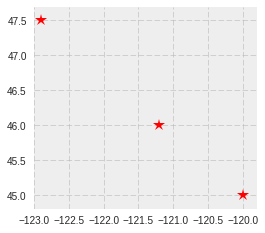Let's get a bit fancier, as a stepping stone to GeoDataFrames. First, we'll define a simple dictionary of lists, that we'll use again later.

In :
data = {'name': ['a', 'b', 'c'],
'lat': [45, 46, 47.5],
'lon': [-120, -121.2, -122.9]}


Note this convenient, compact approach to create a list of Point shapely objects out of X & Y coordinate lists:

In :
geometry = [Point(xy) for xy in zip(data['lon'], data['lat'])]
geometry

Out:
[<shapely.geometry.point.Point at 0x7fbe0437f110>,
<shapely.geometry.point.Point at 0x7fbdd88b1690>,
<shapely.geometry.point.Point at 0x7fbe04359f90>]

We'll wrap up by creating a GeoSeries where we explicitly define the index values.

In :
gs = GeoSeries(geometry, index=data['name'])
gs

Out:
a        POINT (-120 45)
b      POINT (-121.2 46)
c    POINT (-122.9 47.5)
dtype: object

## 4. GeoDataFrames: The real power tool.¶

NOTE/HIGHLIGHT:

• It's worth noting that a GeoDataFrame can be described as a Feature Collection, where each row is a Feature, a geometry column is defined (thought the name of the column doesn't have to be "geometry"), and the attribute Properties are simply the other columns (the Pandas DataFrame part, if you will).
• More than one column can store geometry objects! We won't explore this capability in this tutorial.

We'll build on the GeoSeries examples. Let's reuse the data dictionary we defined earlier, this time to create a DataFrame.

In :
df = pd.DataFrame(data)
df

Out:
lat lon name
0 45.0 -120.0 a
1 46.0 -121.2 b
2 47.5 -122.9 c

Now we use the DataFrame and the "list-of-shapely-Point-objects" approach to create a GeoDataFrame. Note the use of two GeoDataFrame attribute columns, which are just two simple Pandas Series.

In :
geometry = [Point(xy) for xy in zip(df['lon'], df['lat'])]
gdf = GeoDataFrame(df, geometry=geometry)


There's nothing new to visualize, but this time we're using the plot method from a GeoDataFrame, not from a GeoSeries. They're not exactly the same thing under the hood.

In :
gdf.plot(marker='*', color='green', markersize=6, figsize=(2, 2));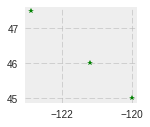### FINALLY, we get to work with real data! Load and examine the simple "oceans" shape file¶

gpd.read_file is the workhorse for reading GIS files. It leverages the fiona package.

In :
oceans = gpd.read_file(os.path.join(data_pth, "oceans.shp"))

In :
oceans.head()

Out:
ID Oceans geometry my_polygon
0 1 South Atlantic Ocean POLYGON ((-67.26025728926088 -59.9309210526315... S.Atlantic
1 0 North Pacific Ocean (POLYGON ((180 66.27034771241199, 180 0, 101.1... N.Pacific
2 3 Southern Ocean POLYGON ((180 -60, 180 -90, -180 -90, -180 -60... Southern
3 2 Arctic Ocean POLYGON ((-100.1196521436255 52.89103112710165... Arctic
4 5 Indian Ocean POLYGON ((19.69705552221351 -59.94160091330382... Indian

The crs was read from the shape file's prj file:

In :
oceans.crs

Out:
{'init': u'epsg:4326'}

Now we finally plot a real map (or blobs, depending on your aesthetics), from a dataset that's global and stored in "geographic" (latitude & longitude) coordinates. It'snot quite the actual ocean shapes defined by coastal boundaries, but bear with me.

In :
oceans.plot();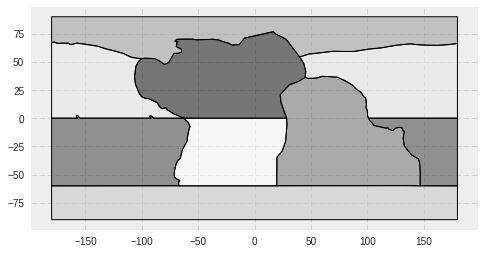oceans.shp stores both Polygon and Multi-Polygon geometry types (but a Polygon may be viewed as a Multi-Polygon with 1 member). We can get at the geometry types and other geometry properties easily.

In :
oceans.geom_type

Out:
0         Polygon
1    MultiPolygon
2         Polygon
3         Polygon
4         Polygon
5    MultiPolygon
6         Polygon
dtype: object
In :
# Beware that these area calculations are in degrees, which is fairly useless
oceans.geometry.area

Out:
0     5287.751094
1    11805.894558
2    10822.509589
3     9578.786157
4     9047.879388
5     9640.457926
6     8616.721287
dtype: float64
In :
oceans.geometry.bounds

Out:
minx miny maxx maxy
0 -71.183612 -60.000000 28.736134 0.000000
1 -180.000000 0.000000 180.000000 67.479386
2 -180.000000 -90.000000 180.000000 -59.806846
3 -180.000000 47.660532 180.000000 90.000000
4 19.697056 -59.945004 146.991853 37.102940
5 -180.000000 -60.000000 180.000000 2.473291
6 -106.430148 0.000000 45.468236 76.644442

The envelope method returns the bounding box for each polygon. This could be used to create a new spatial column or GeoSeries; directly for plotting; etc.

In :
oceans.envelope.plot();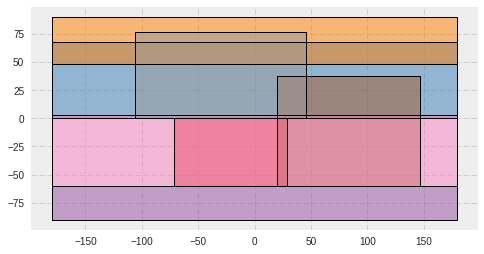Does it seem weird that some envelope bounding boxes, such as the North Pacific Ocean, span all longitudes? That's because they're Multi-Polygons with edges at the ends of the -180 and +180 degree coordinate range.

In :
oceans[oceans['Oceans'] == 'North Pacific Ocean'].plot();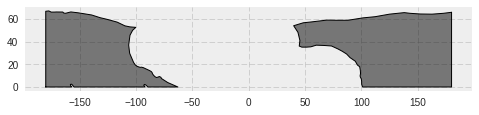### Load "Natural Earth" countries dataset, bundled with GeoPandas¶

"Natural Earth is a public domain map dataset available at 1:10m, 1:50m, and 1:110 million scales. Featuring tightly integrated vector and raster data, with Natural Earth you can make a variety of visually pleasing, well-crafted maps with cartography or GIS software." It (a subset?) comes bundled with GeoPandas and is accessible from the gpd.datasets module. We'll use it as a helpful global base layer map.

In :
world = gpd.read_file(gpd.datasets.get_path('naturalearth_lowres'))

Out:
continent gdp_md_est geometry iso_a3 name pop_est
0 Asia 22270.0 POLYGON ((61.21081709172574 35.65007233330923,... AFG Afghanistan 28400000.0
1 Africa 110300.0 (POLYGON ((16.32652835456705 -5.87747039146621... AGO Angola 12799293.0

Its CRS is also EPSG:4326:

In :
world.crs

Out:
{'init': u'epsg:4326'}
In :
world.plot();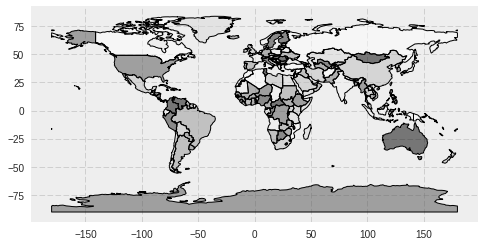### Map plot overlays: Plotting multiple spatial layers¶

Here's a compact, quick way of using GeoDataFrame plot method to overlay two GeoDataFrame, while style customizing the styles for each layer.

In :
world.plot(ax=oceans.plot(cmap='Set2', alpha=1), alpha=1);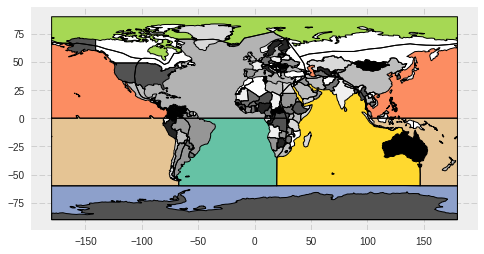NOTE/WATCH:
The oceans polygon boundaries are coming through the world dataset! I've looked into this and can't find a solution. I think it's a bug in the GeoPandas underlying plotting library or plotting approach.

We can also compose the plot using conventional matplotlib steps and options that give us more control.

In :
f, ax = plt.subplots(1, figsize=(10, 5))
# Other nice categorical color maps (cmap) include 'Set2' and 'Set3'
oceans.plot(cmap='Paired', alpha=1, linewidth=0.2, ax=ax)
world.plot(alpha=1, ax=ax)
ax.set_ylim([-100, 100])
ax.set_title('Countries and Ocean Basins')
plt.axis('equal');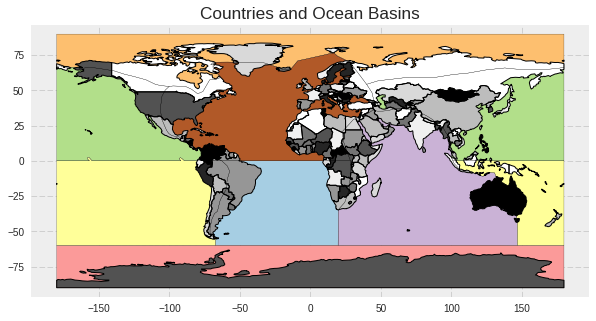## 5. Extras: Reading from other data source types; fancier plotting¶

• Read another shape file and explore filtering and plotting. In the original UW GeoHack Week tutorial, this exercise involved reading geospatial data from a remote PostGIS source.
• Read from a remote OGC WFS service.

### Read from Shapefile with GeoDataFrame¶

In :
seas = GeoDataFrame.from_file(os.path.join(data_pth, "World_Seas.shp"))


Let's take a look at the GeoDataFrame.

In :
seas.head()

Out:
gazetteer geometry id is_generic name oceans
0 4283 POLYGON ((-6.496945454545455 58.08749090909091... 18 False Inner Seas off the West Coast of Scotland North Atlantic Ocean
1 4279 POLYGON ((12.4308 37.80325454545454, 12.414988... 28A False Mediterranean Sea - Western Basin North Atlantic Ocean
2 4280 POLYGON ((23.60853636363636 35.60874545454546,... 28B False Mediterranean Sea - Eastern Basin North Atlantic Ocean
3 3369 POLYGON ((26.21790909090909 40.05290909090909,... 29 False Sea of Marmara North Atlantic Ocean
4 3319 POLYGON ((29.04846363636364 41.25555454545454,... 30 False Black Sea North Atlantic Ocean

### More advanced plotting and data filtering¶

Color the layer based on one column that aggregates individual polygons; using a categorical map, as before, but explicitly selecting the column (column='oceans') and categorical mapping (categorical=True); dispplaying an auto-generated legend; while displaying all polygon boundaries. "oceans" (ocean basins, actually) contain one or more 'seas'.

In :
seas.plot(column='oceans', categorical=True, legend=True, figsize=(14,6));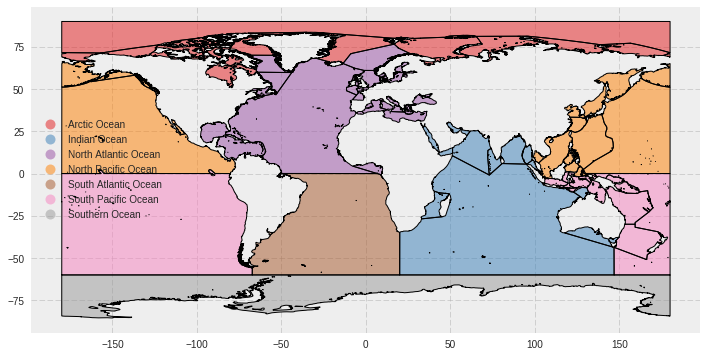NOTE/COOL:
See http://darribas.org/gds15/content/labs/lab_04.html for great examples of lots of other cool GeoPandas map plotting tips.

Combine what we've learned. A map overlay, using world as a background layer, and filtering seas based on an attribute value (from oceans column) and an auto-derived GeoPandas geometry attribute (area). world is in gray scale, while the filtered seas is in color.

In :
seas_na_arealt1000 = seas[(seas['oceans'] == 'North Atlantic Ocean')
& (seas.geometry.area < 1000)]

In :
seas_na_arealt1000.plot(ax=world.plot(alpha=0.1), cmap='Paired')

# Use the bounds geometry attribute to set a nice
# geographical extent for the plot, based on the filtered GDF
bounds = seas_na_arealt1000.geometry.bounds

plt.xlim([bounds.minx.min()-5, bounds.maxx.max()+5])
plt.ylim([bounds.miny.min()-5, bounds.maxy.max()+5]);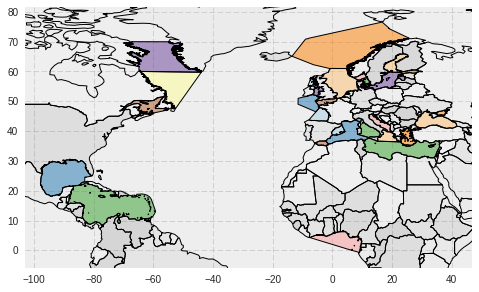### Save the filtered seas GeoDataFrame to a shape file¶

The to_file method uses the fiona package to write to a GIS file. The default driver for output file format is 'ESRI Shapefile', but many others are available because fiona leverages GDAL/OGR.

In :
seas_na_arealt1000.to_file(os.path.join(data_pth, "seas_na_arealt1000.shp"))


### Read from OGC WFS GeoJSON response into a GeoDataFrame¶

Use an Open Geospatial Consortium (OGC) Web Feature Service (WFS) request to obtain geospatial data from a remote source. OGC WFS is an open geospatial standard.

We won't go into all details here about what's going on. Suffice it to say that we issue an OGC WFS request for all features from the layer named "oa:goainv" found in a GeoServer instance from NANOOS, requesting the response in GeoJSON format. Then we "load" it into a geojson feature object (basically a dictionary) using the geojson package.

The "oa:goainv" layer is a global dataset of monitoring sites and cruises where data relevant to ocean acidification is collected. It's a work in progress from the Global Ocean Acidification Observation Network (GOA-ON); for additional information see the GOA-ON Data Portal.

In :
import requests
import geojson

wfs_url = "http://data.nanoos.org/geoserver/ows"
params = dict(service='WFS', version='1.0.0', request='GetFeature',
typeName='oa:goaoninv', outputFormat='json')

r = requests.get(wfs_url, params=params)


Let's examine the general characteristics of this GeoJSON object. We'll take advantage of the __geo_interface__ interface we discussed earlier.

In :
print(type(wfs_geo))
print(wfs_geo.keys())
print(len(wfs_geo.__geo_interface__['features']))

<class 'geojson.feature.FeatureCollection'>
['crs', 'totalFeatures', u'type', 'features']
527


Now we use the from_features constructor method to create a GeoDataFrame, passing to it the features from the __geo_interface__ method.

In :
wfs_gdf = GeoDataFrame.from_features(wfs_geo.__geo_interface__['features'])


Display the values for the last feature, as an example.

In :
wfs_gdf.iloc[-1]

Out:
Oceans                                                     South Pacific Ocean
Source_Doc_kml                                                            None
agency                                       National Science Foundation (NSF)
city
comments                     The Southern Ocean Apex Surface Mooring is co-...
contact_email                                      [email protected]
contact_name
country                                                                     US
cruise_id                                                                  NaN
data_url                     https://rawdata.oceanobservatories.org/files/G...
department
deploy_date
depth_range
duration
frequency                                                           sub-hourly
geometry                                             POINT (-89.2069 -54.4041)
id                                                                         572
latitude                                                              -54.4041
line_xy                                                                   None
location
longitude                                                             -89.2069
method
method_documentation
organization                                    Ocean Observatories Initiative
organization_abbreviation                                                  OOI
overlaps_with
parameters                          temperature; salinity; pH; CO2_air; CO2_sw
parameters_planned
platform_name                GS01SUMO: Global Southern Ocean Apex Surface M...
platform_name_kml                                                         None
platform_type                                                                M
point_xy                                                                  None
project
sensors
source_doc                                                                 OOI
track_pt_lat                                                              None
track_pt_lon                                                              None
type                                                      Apex Surface Mooring
url                          http://oceanobservatories.org/site/gs01sumo/; ...
Name: 526, dtype: object

Finally, a simple map overlay plot.

In :
wfs_gdf.plot(ax=world.plot(alpha=1), figsize=(10, 6),
marker='o', color='red', markersize=4);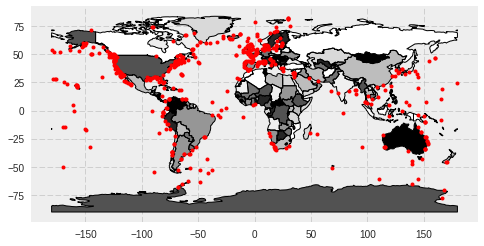In [ ]: